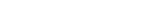MathExamle.com# Parentheses

Select from the list a generator that creates examples that can contain parentheses

Creates examples with addition and subtraction operations of integers, the answers are always positive

5 Variants 5 Levels
 36-22= 28+52= 49+14= 49-29= 48-4= 56+0=
Variant: 3 Level: Normal

Creates examples with multiplication and division integers

6 Variants 4 Levels
 4×10= 11×9= 5×4= 2×3= 8×6= 0×3= 38×2= 3×0=
Variant: 1 Level: Easy

Creates examples with operations of addition, subtraction, multiplication and division of integers, the answers are always positive

3 Variants 3 Levels
 687-6×102= 538+13×18= 36×10-294= 30×10+498=
Variant: 1 Level: Normal

### Conversant

Creates examples with addition and subtraction operations of integers, the answers and intermediate results can be negative

4 Variants 5 Levels
 303217-(0-660000)= -270000-29000-1000=
Variant: A Level: Hard

### Partitive

Creates examples with addition and subtraction operations of decimals, the answers are always positive

3 Variants 4 Levels
 132.218+120+37.782= 100+282.387-273.755=
Variant: 2 Level: Hard

Creates examples with multiplication and division of decimals

5 Variants 4 Levels
 22.44÷1.6= 24.48÷3.2= 1.125÷3= 3÷1.875=
Variant: 2 Level: Difficult

### Numerator

Creates examples with addition and subtraction of fractions, the answers are always positive

3 Variants 3 Levels
 1 + 7 – 2 = 5 10 5
 2 + ( 3 – 1 ) = 5 5 4
Variant: A Level: Easy

Creates examples with operations of addition, subtraction, multiplication and division of integers, the answers can be negative

3 Variants 3 Levels
 23+3×19= -20+5×2= -24×2-35= -2×4+27= -96÷6-40= -29-24÷12=
Variant: 1 Level: Easy

Creates examples with operations of multiplication and division of negative and positive integers

4 Variants 4 Levels
 -210÷(6÷(-3))= 7×2×(-20)= 760÷40= 320÷(16÷2)÷10=
Variant: A Level: Normal

Creates examples with addition and subtraction operations of decimals, the answers can be negative

3 Variants 4 Levels
 51+45= 16.7+5= 33.7-5.7= -79.5+64= -84-14.4= 91+3=
Variant: 1 Level: Medium

Creates examples with operations of addition, subtraction, multiplication and division of decimals, the answers are always positive

3 Variants 4 Levels
 11.1+3.8×3= 96÷3.2-9.3= 1÷0.5+59.3= 27÷1.5-16.9=
Variant: 1 Level: Easy

Creates examples with operations of addition, subtraction, multiplication and division of decimals, the answers can be negative

3 Variants 4 Levels
 50.5-(0.652+0.158)×50= -87.084+16.416÷72÷5.7=
Variant: A Level: Difficult

### Denominator

Creates examples with addition and subtraction of fractions, the answers can be negative

3 Variants 3 Levels
 – 1 + 6 – 4 = 5 7 5
 – 9 + 3 + 1 = 10 10 10
Variant: 2 Level: Easy

Creates examples with multiplication and division of fractions

3 Variants 3 Levels
 1 1 × 2 = 2 3
 1 × ( 4 ÷ 1 ) = 2 3 9
Variant: A Level: Easy

Creates examples with operations of addition, subtraction, multiplication and division of fractions, the answers are always positive

2 Variants 3 Levels
 17 8 + 11 × 52 = 15 13 55
Variant: A Level: Hard

Creates examples with operations of addition, subtraction, multiplication and division of fractions, the answers can be negative

2 Variants 3 Levels
 22 × 56 + 273 = 119 11 17
 105 × 368 – 8 = 152 735 133
Variant: 1 Level: Hard